# Background

Randomization-Based Inference is a method used to determine the causal effect of treatments on outcomes. This method can be used to compute p-values, obtain Fisher Intervals, retrieve counternull sets, and adjust p-values. Unlike traditional Frequentist methods, this approach does not rely on any distributional assumptions on the data. Thus, this method may be used on data that does not follow a normal distribution.

Randomization-Based Inference utilizes the Neyman-Rubin potential outcomes notation (Pearce S.C., 1992). Here we specify N experimental units (indexed by i) that receive either an active treatment, Wi = 1, or a control treatment, Wi = 0. We define the outcomes of each experimental unit as a function of the treatment. Yi(Wi = 1) and Yi(Wi = 0) corresponds to the outcome of experimental unit i when exposed to an active treatment and control treatment respectively.

# Test Statistics and Fisher-Exact P-Values

Consider a study conducted on 156 fish. Half of the fish are exposed to flashing lights and the other half are not. We will use Randomization-Based Inference to determine the effect that the flashing lights had on the movement of the fish.

library(Counternull)

y = sample_data$turn_angle # fish turn angles w = sample_data$w # treatment assignments (1 = exposed, 0 = control)
test_stat = "diffmeans" # average difference in the turn angles between the
# two groups of fish
cat(find_test_stat(y, w, test_stat))
#> 3.091943

Here, our outcome (y) is the angle in which the fish turn when swimming. The treatment assignments (w) indicate whether or not a fish was exposed to flashing lights.

We choose to evaluate the difference of means test statistic. However, we have the choice to use any test statistic including those without known variances or those we come up with on our own. In this case, the difference of means test statistic tell us that there was a 3.09 degree increase in the mean turn angles of the fish exposed to flashing lights compared to those that were not.

Now we would like to compute p-values for our data.

n_rand = create_null_rand(y, w, sample_matrix, test_stat) # obtain "null_rand"
# object
summary(n_rand)
#> Observed test statistic: 3.091943
#> Number of extreme test statistics: 56
#> P-value: 0.056
#> Alternative: two-sided
plot(n_rand)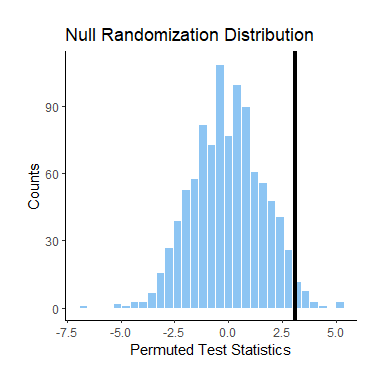The above code constructs a null randomization distribution. This is done by computing a test statistic for each assignment permutation specified in “sample_matrix”. This distribution relies on the sharp null hypothesis: Yi(Wi=1) - Yi(Wi=0) = 0. This is the assumption that the treatments have no effect on the outcomes. In this case, this means that we assume the turn angle of a fish is the same whether or not that fish was exposed to flashing lights.

We find the Fisher-Exact P-Value to be .056. This is equivalent to the proportion of test statistics in the distribution that are as or more extreme than the observed test statistic (3.09). Because our alternative is two-sided, “extreme” is defined as test statistics greater or equal to the absolute value of the observed test statistic.

print(sample_matrix[1:10,1:2])
#>    V1 V2
#> 1   0  0
#> 2   1  1
#> 3   0  0
#> 4   1  0
#> 5   1  1
#> 6   1  1
#> 7   1  0
#> 8   0  1
#> 9   0  1
#> 10  0  1

Here, we see an example of the treatment assignments for the first two permutations in “sample_matrix” for the first 10 fish. Each permutation is unique and is what is used to generate the null randomization distribution.

# Counternull Values

Next, we will compute counternull values for our data. Counternull values are effect sizes that have the same evidence as the sharp null hypothesis (Rosenthal and Rubin 1994). They provide an alternate assumption to evaluate our data.

Counternull distributions are constructed using the sharp counternull hypothesis: Yi(Wi=1) - Yi(Wi=0) = a, where a is a counternull value. This hypothesis assumes the difference in the outcomes of the experimental units when they are exposed to different treatments is equal to the counternull value.

All possible values for a are within the counternull set of the data. These values produce counternull randomization distributions with the same Fisher-Exact P-Value as the null randomization distribution.

The Fisher-Exact P-Value from the counternull randomization distribution is the proportion of test statistics in the distribution that are as or more extreme than the observed test statistic (3.09). However, more extreme is defined in the opposite direction as in the null randomization distribution. So now, we look at test statistics less than or equal to the absolute value of 3.09.

c_value = find_counternull_values(n_rand)
summary(c_value)
#> Counternull Set (Positive): [ 5.782512 , 5.817145 ]
#> Counternull Set (Negative): [ -5.851778 , -5.841883 ]
plot(c_value)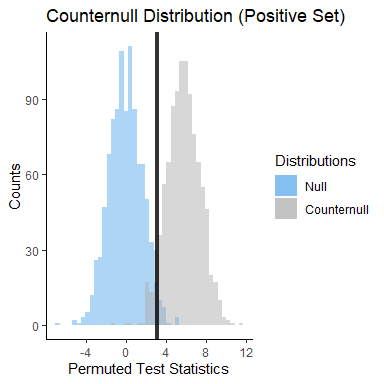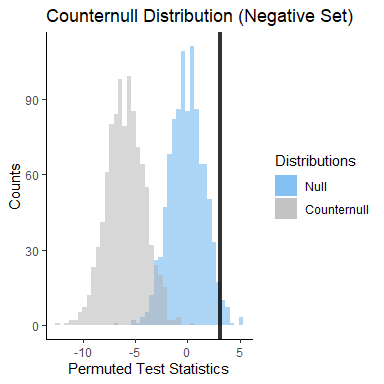If we recall from earlier, our Fisher-Exact P-Value from the data is .056. While a p-value larger than .05 may tempt us to claim that flashing lights have no effect on fish turn angles, our counternull sets remind us not to do so.

We retrieve two counternull sets. This means that there is the same amount of evidence to support the null effect (no change in turn angles due to flashing lights) as there is to support a change of 5 degrees in the mean turn angles as a result of flashing lights. If we are willing to claim that flashing lights had no effect on fish turn angles, we have to be equally willing to claim the lights had an effect equivalent to a 5 degree change in the average turn angles of the fish.

Thus, the counternull sets prevents us from incorrectly accepting the null hypothesis and provides an alternative hypothesis to examine the data.

Additionally, if would like to round our p-value from .056 to .06, we can retrieve the counternull set corresponding to the rounded p-value. We do this by specifying the “counts” parameter.

c_value = find_counternull_values(n_rand, c(55,60))
summary(c_value)
#> Counternull Set (Positive): [ 5.720667 , 5.822092 ]
#> Counternull Set (Negative): [ -5.861673 , -5.824566 ]
plot(c_value)Since there are 1,000 test statistics in the distribution, counts 55-60 correspond to p-values that round to .06 (.055-.06).

# Fisher Intervals

Fisher or Fidicual Intervals are a range of effect sizes that are consistent with data at a specified confidence level (Zabrocki 2021). They are analogous to Confidence Intervals used in a Frequentist setting. Unlike traditional Confidence Intervals, Fisher Intervals (FI) do not require data to be normally distributed and report unit-level effect sizes.

fisher = create_fisher_interval(n_rand)
summary(fisher)
#> Fisher Interval: [ -0.06802955 , 6.450438 ]
#> Alpha: 0.05
#> P-Value Lower: 0.025 P-Value Upper: 0.975
plot(fisher)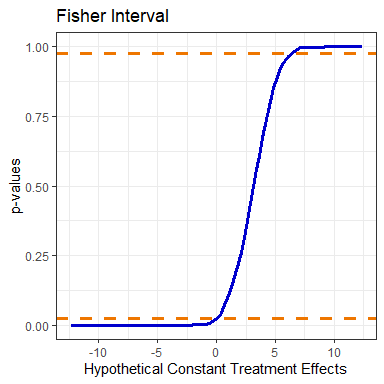t.test(sample_data$turn_angle[w == 1], sample_data$turn_angle[w == 0],
conf.level = 0.95)$conf.int #>  -0.1564843 6.3403702 #> attr(,"conf.level") #>  0.95 Here we can compare the results obtained from the Fisher Interval to the traditional confidence interval. # Fisher-Adjusted P-Values and Randomization Matrices Say we want to compute multiple comparisons on the same dataset. We can use a randomization-based method to adjust our p-values for multiple testing (Lee et al., 2017). Unlike Bonferroni adjustments, this method accounts for correlations within the data resulting in higher power. First, we look at a second comparison with our data. This time we will compare the distributions of the two groups of fish using a Kolmogorov Smirnov test. We will also create a new randomization matrix. fun = function(x,y){ # write a function to compute KS test return(invisible(ks.test(x,y)$statistic))
}

rand_matrix = create_randomization_matrix(156,1000) # create rand matrix
# 156 fish, 1000 permutations

n_rand_two = create_null_rand(y, w, rand_matrix, fun = fun,
alternative = "greater")
summary(n_rand_two)
#> Observed test statistic: 0.2948718
#> Number of extreme test statistics: 4
#> P-value: 0.004
#> Alternative: greater
plot(n_rand_two)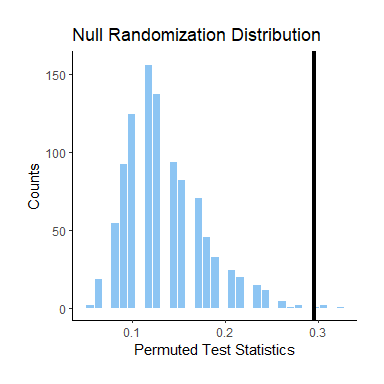In this example, we use 1,000 permutations in our randomization matrix. But how do you determine the ideal amount of permutations to use? If your data set is small enough, then you can use the maximum number of permutations.

For example, if you have 6 experimental units in your study with half of them assigned to either an active treatment or control, then there are only $$\binom{6}{3}$$ possible permutations to include in the randomization matrix.

With larger data sets, there is a trade off between accuracy and efficiency when using larger amounts of permutations. If you would like more significant digits for your p-value, then consider increasing the number of permutations in your randomization matrix. If increasing the number of permutations does not change the shape of the null randomization distribution and does not substantially change the p-value, then you may prefer to use less permutations.

adjusted_pvalues = adjust_pvalues(list(n_rand,n_rand_two))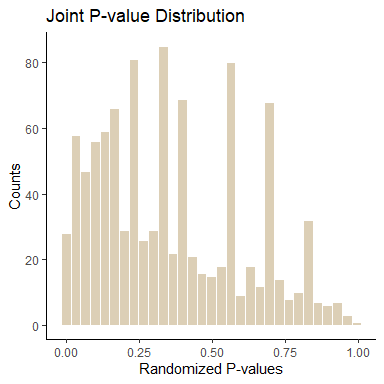cat(adjusted_pvalues)
#> 0.093 0.008

Now, we have adjusted our p-values. Here we can visualize the joint p-value distribution from our two comparisons as well as the adjusted p-values.

# Conclusion

Randomization-Based Inference allows us to draw conclusions on the effects of treatments on outcomes. We avoid making distributional assumptions which allows us to work with data of smaller sample sizes. Additionally, this method gives us the choice to use any test statistic including those with unknown variances.

Reporting counternull values is a good practice to prevent common mistakes in hypothesis testing. Finally, Fisher-Adjusted P-Values are a powerful way to implement multiple testing while accounting for correlation within data.